Constant Resonance Gain Next  |  Prev  |  Up  |  Top  |  Index  |  JOS Index  |  JOS Pubs  |  JOS Home  |  Search

Constant Resonance Gain

It turns out it is possible to normalize exactly the resonance gain of the second-order resonator tuned by a single coefficient . This is accomplished by placing the two zeros at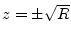, where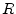is the radius of the complex-conjugate pole pair . The transfer function numerator becomes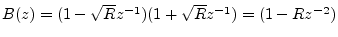, yielding the total transfer function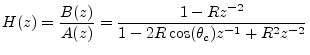which corresponds to the difference equation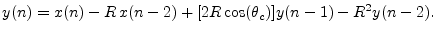We see there is one more multiply-add per sample (the term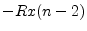) relative to the unnormalized two-pole resonator of Eq. (10.13). The resonance gain is now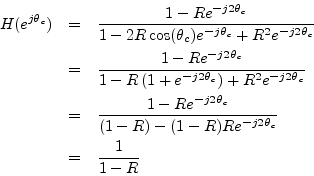Thus, the gain at resonance is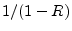for all resonance tunings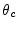.

Figure 10.20 shows a family of amplitude responses for the constant resonance-gain two-pole, for various values ofand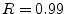. We see an excellent improvement in the regularity of the amplitude response as a function of tuning.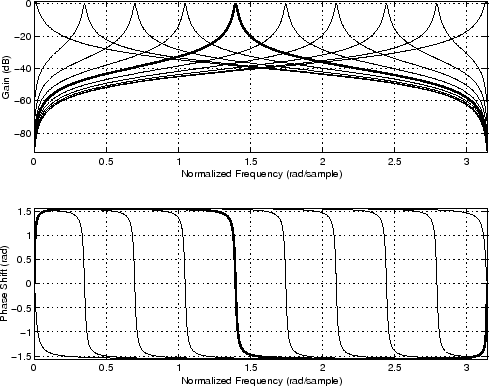Next  |  Prev  |  Up  |  Top  |  Index  |  JOS Index  |  JOS Pubs  |  JOS Home  |  Search

[How to cite this work] [Order a printed hardcopy]